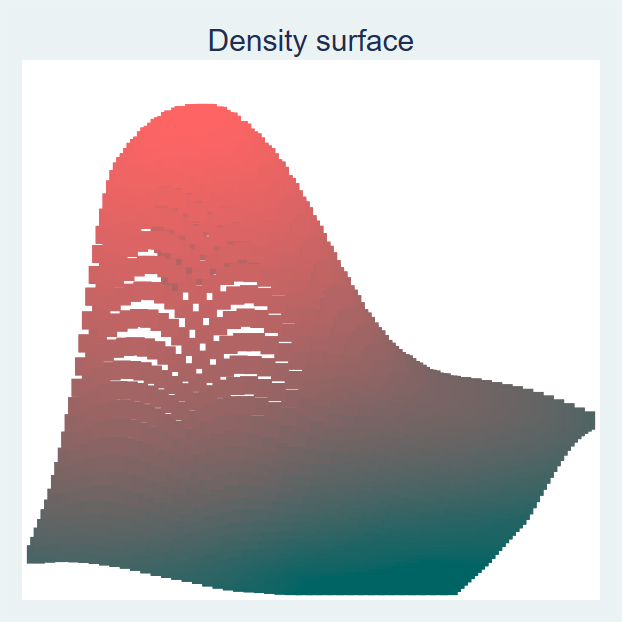Search

# A Bivariate Kernel Density Graph in Stata

You can create three types of bivariate kernel density plots in Stata, one is a 2D heatmap, the second is a surface plot, and the third is a bar plot.

To create these graphs in Stata, you first need to download the command tddens from the SSC, which you do in Stata using the following command:To generate all three of these graphs in Stata, use the following commands:

The first graph is always generated by the tddens command, the second graph is generated using the sgraph option, and the third graph is generated using the bgraph option.